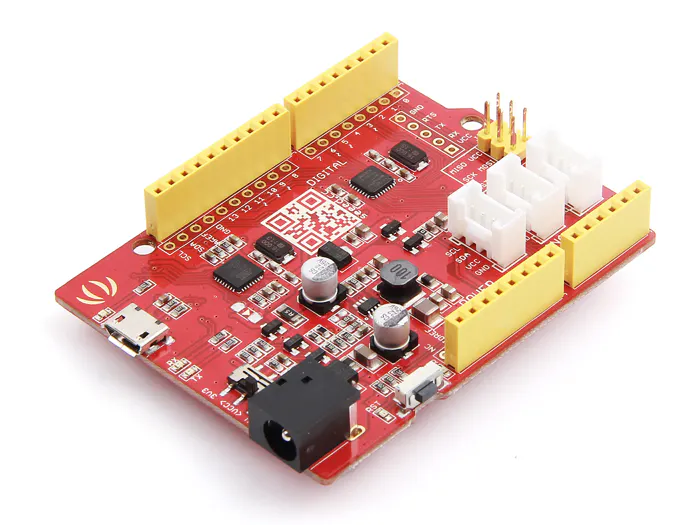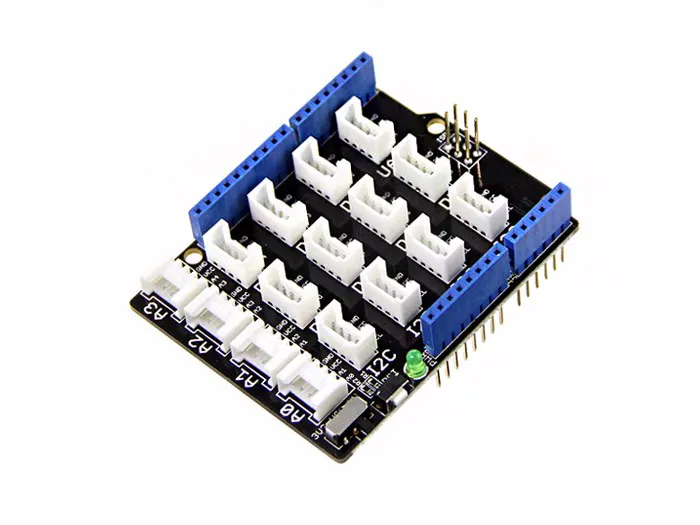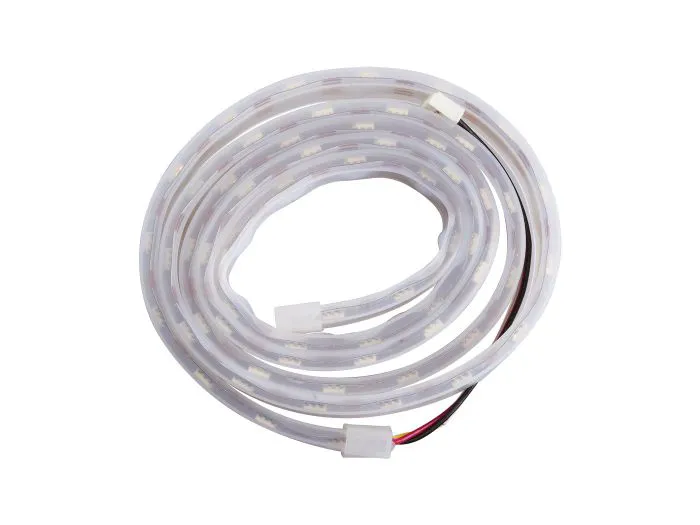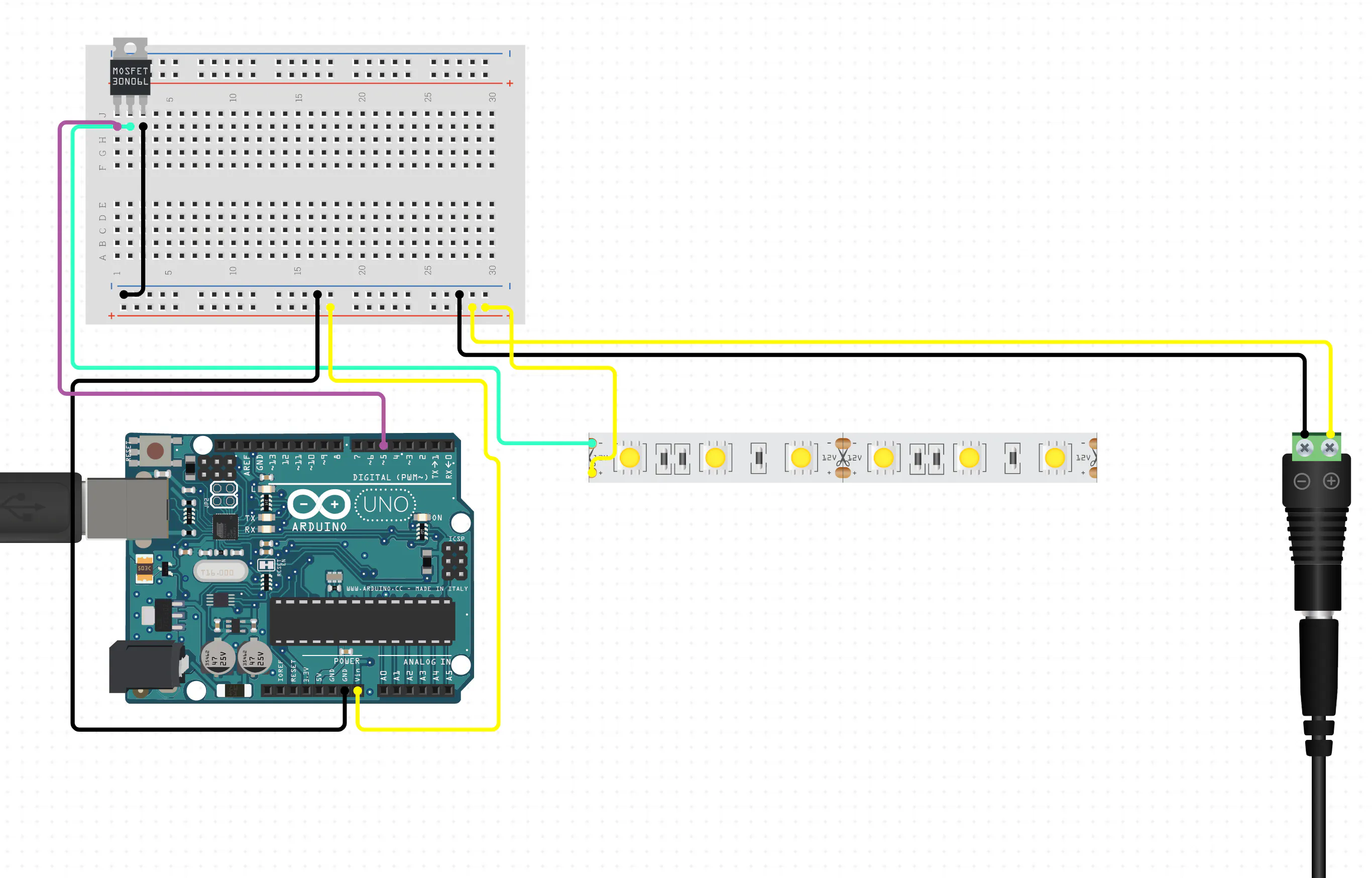# Visualize sorting algorithms with Arduino and LED strip

Feel confused about sorting algorithms? Use LEDs and Arduino to get better understanding about them!

BeginnerFull instructions provided1 hour501

## Things used in this project

### Hardware componentsSeeeduino V4.2
×1Seeed Base Shield V2
×1Seeed Waterproof WS2813 RGB LED Strip Waterproof
×1

### Software apps and online servicesArduino IDE

## Schematics

### Hardware Schematic

Remember to connect your led strip to the right digital pin!## Code

### Bubble sort

Arduino
Remember to set digital pin number and number of leds correctly
```#include <FastLED.h>

#include "SparkFunLSM6DS3.h"
#include "Wire.h"
#include "math.h"

// Which pin on the Arduino is connected to the NeoLEDS?
#define NUM_LEDS 30
#define DATA_PIN 6
CRGB leds[NUM_LEDS];

int r, g, b;

void setup() {
Serial.begin(9600);
FastLED.setBrightness(100); //init led strip
}

void loop() {
bubbleSort(); //call bubbleSort function
exit(0); //stop loop
}

void bubbleSort()
{
int arr[NUM_LEDS]; //init an array with 30 elements
for (int j = 0; j < NUM_LEDS; j++)
{
int i = random(NUM_LEDS);
arr[j] = i;
setColor(i, j);
}
/*
give every led a randnom number between (0,30). The number will be used to generate a unique color to represent the value of the number
*/

for (int m = 0; m < NUM_LEDS - 1; m++) {
for (int n = 0; n < NUM_LEDS - 1 - m; n++) {
if (arr[n] > arr[n + 1]) {
setColor(arr[n + 1], n); // swap the larger number and smaller number
setColor(arr[n], n + 1);
int temp = arr[n + 1];
arr[n + 1] = arr[n];
arr[n] = temp;
}
// bubble sort algorithm
}
}
}
void setColor(int color, int led) {
// use an int number between (0,30) to determine the color of the specified led
if (color < NUM_LEDS / 3) {
r = 255;
g = floor(250 * 3 * color / NUM_LEDS);
b = 0;
} else if (color < NUM_LEDS / 2) {
r = floor(750 - color * (250 * 6 / NUM_LEDS));
g = 255;
b = 0;
} else if (color < NUM_LEDS * 2 / 3) {
r = 0;
g = 255;
b = floor(color * (250 * 6 / NUM_LEDS) - 750);
} else if (color < NUM_LEDS * 5 / 6) {
r = 0;
g = floor(1250 - color * (250 * 6 / NUM_LEDS));
b = 255;
} else {
r = floor(150 * color * (6 / NUM_LEDS) - 750);
g = 0;
b = 255;
}
leds[led].setRGB(g, r, b);
FastLED.show(); // This sends the updated piaXel color to the hardware.
delay(30);
}
```

### Quick sort

Arduino
Remember to set the data pin and number of leds correctly
```#include <FastLED.h>

#include "SparkFunLSM6DS3.h"
#include "Wire.h"
#include "math.h"

// Which pin on the Arduino is connected to the NeoLEDS?
// On a Trinket or Gemma we suggest changing this to 1
#define NUM_LEDS 30
#define DATA_PIN 6
CRGB leds[NUM_LEDS];

int r, g, b;

void setup()
{
Serial.begin(9600);
FastLED.setBrightness(100); //init the led strip with 100 brightness
}

void loop()
{
int arr[NUM_LEDS];
for (int j = 0; j < NUM_LEDS; j++)
{
int i = random(NUM_LEDS);
arr[j] = i;
setColor(i, j);
} //set the led strip with 30 random unique color representing different values
quickSort(arr, 0, 29); // call quicksort function
exit(0);
}

void quickSort(int num[], int l, int r)
{
if (l >= r)
return;
int m = l, n = r, x = num[l];
while (m < n)
{
while (m < n && num[n] >= x)
n--;
if (m < n)
{
setColor(num[n], m);
num[m++] = num[n];
}

while (m < n && num[m] <= x)
m++;
if (m < n)
{
setColor(num[m], n);
num[n--] = num[m];
}
}
num[m] = x;               //put the inital x into m element
setColor(x, m);
quickSort(num, l, m - 1); //use m as mid value, use recursive call
quickSort(num, m + 1, r);
}
void setColor(int color, int led)
{
if (color < NUM_LEDS / 3)
{
r = 255;
g = floor(250 * 3 * color / NUM_LEDS);
b = 0;
}
else if (color < NUM_LEDS / 2)
{
r = floor(750 - color * (250 * 6 / NUM_LEDS));
g = 255;
b = 0;
}
else if (color < NUM_LEDS * 2 / 3)
{
r = 0;
g = 255;
b = floor(color * (250 * 6 / NUM_LEDS) - 750);
}
else if (color < NUM_LEDS * 5 / 6)
{
r = 0;
g = floor(1250 - color * (250 * 6 / NUM_LEDS));
b = 255;
}
else
{
r = floor(150 * color * (6 / NUM_LEDS) - 750);
g = 0;
b = 255;
}
// convert an int value to rgb array
leds[led].setRGB(g, r, b);
FastLED.show(); // This sends the updated piaXel color to the hardware.
delay(30);
}
```

## Credits

### memoryleak

5 projects • 5 followers
A soft and hardware engineer motivated by interest and fun